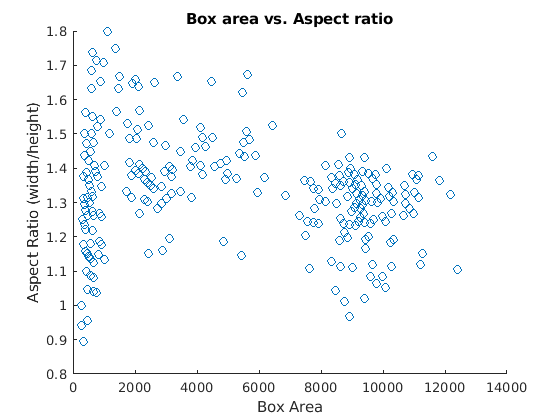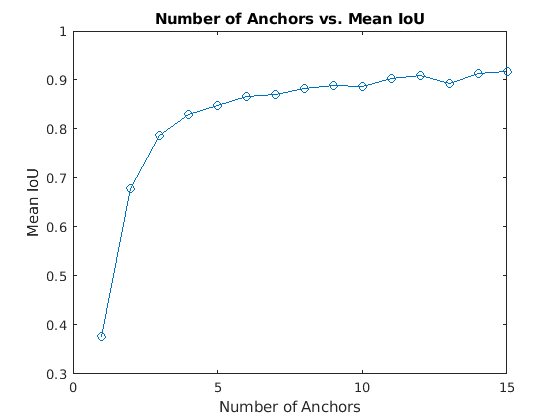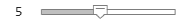Documentation

### This is machine translation

Mouseover text to see original. Click the button below to return to the English version of the page.

Note: This page has been translated by MathWorks. Click here to see
To view all translated materials including this page, select Country from the country navigator on the bottom of this page.

## Estimate Anchor Boxes Using Clustering

This example shows how to estimate anchor boxes from object detector training data using clustering .

Anchor boxes are important parameters of deep learning object detectors such as Faster R-CNN and YOLO v2. The shape, scale, and number of anchor boxes impact the efficiency and accuracy of the detectors. This example shows how to estimate anchor boxes from a vehicle detector training dataset using the K-Medoids clustering algorithm.

See Anchor Boxes for Object Detection to learn more about anchor boxes.

### Load Training Data

Load the vehicle dataset, which contains 295 images and associated box labels.

```% Load vehicle training data. data = load('vehicleTrainingData.mat'); vehicleDataset = data.vehicleTrainingData; % Add fullpath to the local vehicle data folder. dataDir = fullfile(toolboxdir('vision'),'visiondata'); vehicleDataset.imageFilename = fullfile(dataDir, vehicleDataset.imageFilename); % Display dataset summary summary(vehicleDataset)```
```Variables: imageFilename: 295×1 cell array of character vectors vehicle: 295×1 cell ```

### Visualize Ground Truth Box Distribution

Visualize the labeled boxes to better understand the range of object sizes present in the dataset.

```% Combine all the ground truth boxes into one array. allBoxes = vertcat(vehicleDataset.vehicle{:});```

Plot the box area versus the box aspect ratio.

```% Plot the box area versus box aspect ratio. aspectRatio = allBoxes(:,3) ./ allBoxes(:,4); area = prod(allBoxes(:,3:4),2); figure scatter(area,aspectRatio) xlabel("Box Area") ylabel("Aspect Ratio (width/height)"); title("Box area vs. Aspect ratio")```Visually, you see a few groups of objects that are of similar size and shape, but the groups are spread out. This makes it difficult to manually choose anchor boxes. A better way to estimate anchor boxes is to use a clustering algorithm that can group similar boxes together using a meaningful metric.

### Cluster Ground Truth Boxes

Cluster the boxes using the `kmedoids` function with custom intersection-over-union (IoU) distance metric. Other clustering functions such as `clusterdata` or `dbscan` may also be used.

A distance metric based on IoU is invariant to the size of boxes, unlike the Euclidean distance metric, which produces larger errors as the box sizes increase . In addition, an IoU distance metric leads to boxes of similar aspect ratio and sizes being clustered together, which results in anchor box estimates that fit the data. The IoU distance metric is implemented in the supporting function, `iouDistanceMetric`.

Select the number of anchors and estimate the anchor boxes using `kmedoids`. The cluster centers returned by `kmedoids` are the anchor box estimates.

```% Select the number of anchor boxes. numAnchors = 4; % Cluster using K-Medoids. [clusterAssignments, anchorBoxes, sumd] = kmedoids(allBoxes(:,3:4),numAnchors,'Distance',@iouDistanceMetric); % Display estimated anchor boxes. The box format is the [width height]. anchorBoxes```
```anchorBoxes = 4×2 59 43 22 18 29 23 109 84 ```
```% Display clustering results. figure gscatter(area,aspectRatio,clusterAssignments); title("K-Mediods with "+numAnchors+" clusters") xlabel("Box Area") ylabel("Aspect Ratio (width/height)"); grid```Choosing the number of anchors is another training hyperparameter that requires careful selection using empirical analysis. One quality measure for judging the estimated anchor boxes is the mean IoU of the boxes in each cluster. Calculate this using the cluster assignments produced by `kmedoids`.

```% Count number of boxes per cluster. Exclude the cluster center while % counting. counts = accumarray(clusterAssignments, ones(length(clusterAssignments),1),[],@(x)sum(x)-1); % Compute mean IoU. meanIoU = mean(1 - sumd./(counts))```
```meanIoU = 0.8244 ```

The mean IoU should be greater than 0.5 to ensure anchor boxes overlap well with the boxes in the training data. Increasing the number of anchors may improve the mean IoU measure. However, using more anchor boxes in an object detector may increase the computation cost and lead to overfitting, which results in poor detector performance.

Sweep over a range of values and plot the mean IoU versus number of anchor boxes to measure the trade-off between number of anchors an mean IoU.

```maxNumAnchors = 15; for k = 1:maxNumAnchors % Estimate anchors using clustering. [clusterAssignments, anchorBoxes, sumd] = kmedoids(allBoxes(:,3:4),k,'Distance',@iouDistanceMetric); % Compute mean IoU. counts = accumarray(clusterAssignments, ones(length(clusterAssignments),1),[],@(x)sum(x)-1); meanIoU(k) = mean(1 - sumd./(counts)); end figure plot(1:maxNumAnchors, meanIoU,'-o') ylabel("Mean IoU") xlabel("Number of Anchors") title("Number of Anchors vs. Mean IoU")```Two anchor boxes provide a mean IoU above 0.7 and there is marginal improvement in mean IoU beyond 6 anchor boxes. Given these results, the next step is to train and evaluate multiple object detectors using values between 2 and 6. This empirical analysis helps determine the number of anchor boxes required to satisfy application performance requirements such as detection speed or accuracy.

### References

1. Redmon, Joseph, and Ali Farhadi. "YOLO9000: Better, Faster, Stronger." 2017 IEEE Conference on Computer Vision and Pattern Recognition (CVPR). IEEE, 2017.

### Supporting Functions

```function dist = iouDistanceMetric(boxWidthHeight,allBoxWidthHeight) % Return the IoU distance metric. The bboxOverlapRatio function % is used to produce the IoU scores. The output distance is equal % to 1 - IoU. % Add x and y coordinates to box widths and heights so that % bboxOverlapRatio can be used to compute IoU. boxWidthHeight = prefixXYCoordinates(boxWidthHeight); allBoxWidthHeight = prefixXYCoordinates(allBoxWidthHeight); % Compute IoU distance metric. dist = 1 - bboxOverlapRatio(allBoxWidthHeight, boxWidthHeight); end function boxWidthHeight = prefixXYCoordinates(boxWidthHeight) % Add x and y coordinates to boxes. n = size(boxWidthHeight,1); boxWidthHeight = [ones(n,2) boxWidthHeight]; end```

Download ebook